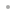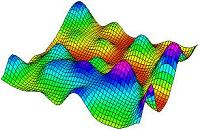MathOptimizerFeaturesReferencesGetting StartedUser GuideBuy Online# MathOptimizer

### An Advanced Modeling and Optimization System for Mathematica UsersMathOptimizer enables the global and local numerical solution of a very general class of optimization problems defined by a finite number of real-valued, continuous functions over a finite n-dimensional interval region.

Special emphasis is placed on nonlinear models, including those that typically have an unknown number of local optima. Nonlinear and global optimization problems are ubiquitous in the sciences, engineering and economics. Several prominent examples are systems of nonlinear equations and inequalities, nonlinear regression, forecasting models, data classification, minimal-energy models, various packing problems, risk management and other stochastic decision problems, and the design and operation of "black box" engineering systems (which are often defined by a complicated, numerically intensive procedure).

MathOptimizer consists of two core solver packages and a solver integrator package. The first core solver package is used for approximate global optimization of an aggregated merit (exact penalty) function on a given interval range. This package is based on a globally convergent adaptive stochastic search procedure, and it also incorporates statistical estimation techniques.

The second core solver package is meant for precise local optimization. It is based on the standard nonlinear (convex) programming approach and refines a given initial solution. The solver integrator package supports the individual or combined use of the core solver packages, but both of the core packages can also be used in standalone mode.

The MathOptimizer User Guide includes concise mathematical background notes and useful modeling tips. It also discusses a number of test problems and several nontrivial application examples. The guide can be accessed directly through Mathematica's online help system.